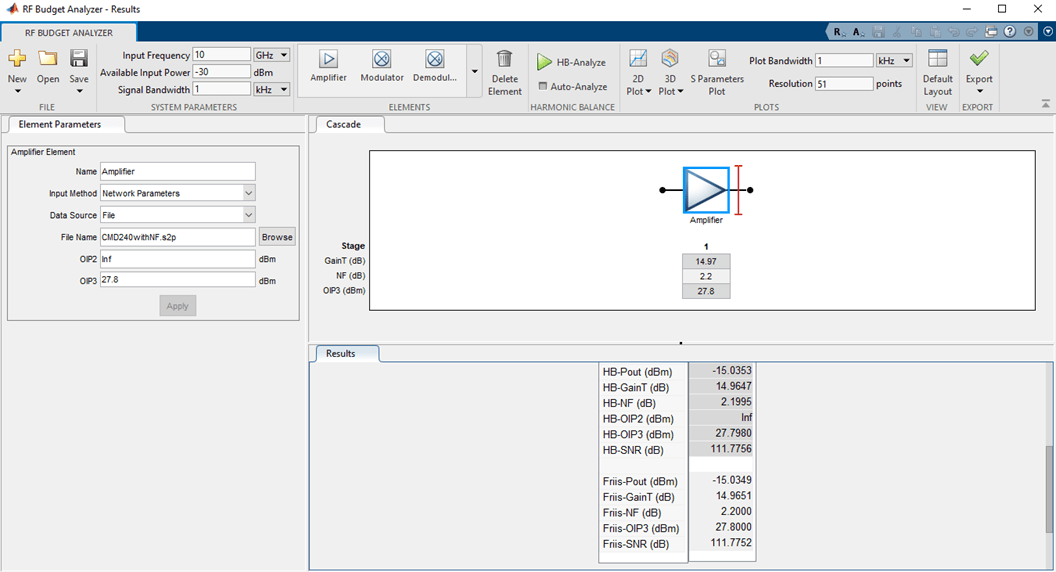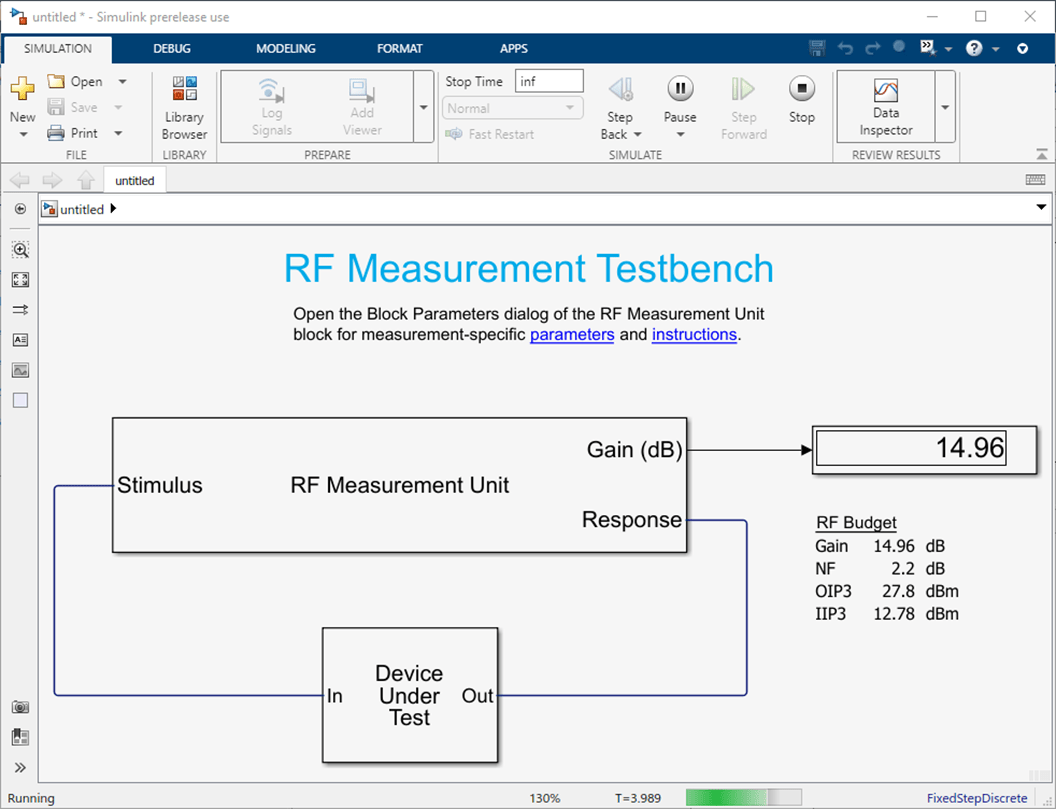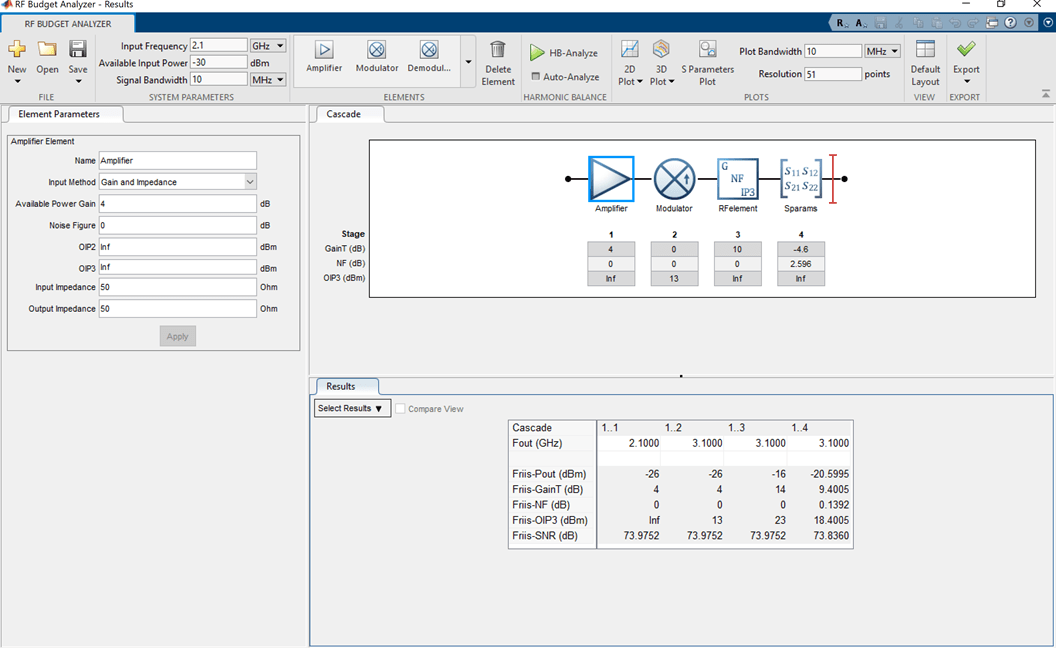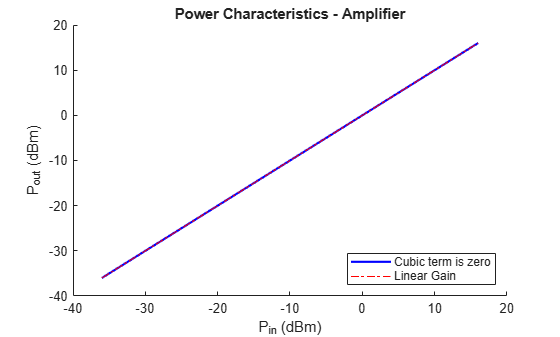# amplifier

Create two-port amplifier element

## Description

Use the `amplifier` object to create a two-port amplifier element or to analyze a commercial off-the-shelf (COTS) amplifier. You can also use the `amplifier` object to model an amplifier in an RF system created using an `rfbudget` object or the RF Budget Analyzer app and, then export this element to RF Blockset™ or to `rfsystem` System object™ for circuit envelope analysis.

## Creation

### Syntax

``amp = amplifier``
``amp = amplifier(Name=Value)``

### Description

example

````amp = amplifier` creates an amplifier object with default property values. ```

example

````amp = amplifier(Name=Value)` sets amplifier object properties using one or more name-value arguments. You can specify multiple name-value arguments.```

## Properties

expand all

Name of amplifier, specified as a character vector. All names must be valid MATLAB® variable names.

Example: `Name='amp'`

S-parameter characterization in amplifier, specified as a logical `true` or `1` or `false` or `0`. When you set this property to `true`, the `amplifier` object uses the `NetworkData` and `NoiseData` properties to compute gain, matching, and noise. When you set this property to `false`, the `amplifier` object use the `Gain`, `Zin`, `Zout`, and `NF` properties to compute gain, matching, and noise.

Example: `UseSparameters=1`

Available power gain, specified as a real finite scalar in dB.

Example: `Gain=10`

Noise figure, specified as a real finite nonnegative scalar dB.

Example: `NF=-10`

Second-order output-referred intercept point, specified as a real scalar in dBm.

Example: `OIP2=8`

Third-order output-referred intercept point, specified as a real scalar in dBm.

Example: `OIP3=10`

Input impedance, specified as a positive real part finite scalar in ohms. You can also use a complex value with a positive real part.

Example: `Zin=40`

Output impedance, specified as a scalar in ohms. You can also use a complex value with a positive real part.

Example: `Zout=40`

Name of the two-port Touchstone file from which to extract the `NetworkData` and the `NoiseData` properties, specified as a string scalar or character vector.

Example: `FileName='default.s2p'`

Network data of the amplifier, specified as a two-port network parameter object. The network parameter objects are of the type:

Network parameter object defines the frequency-dependent gain and impedance matching for the amplifier, typically, a `sparameters` object from a two-port Touchstone file. To specify a frequency-independent network data, set the `NetworkData` property to `[]`. This resets network data to a frequency-independent two-port network object defined by the `Gain`, `Zin`, and `Zout` properties.

Example: `NetworkData=nd`

Noise data of the amplifier, specified as a `noiseParameters` object. The `noiseParameter` object contains a frequency-dependent noise figure loaded from a two-port Touchstone file or built at the MATLAB® command line. To specify a frequency-independent noise figure, set the `NoiseData` to `[]`.

Example: `NoiseData=np`

Number of ports, returned as a scalar integer.

Names of port terminals, returned as a cell vector.

## Object Functions

 `clone` Create copy of existing circuit element or circuit object `rfplot` Plot cumulative RF budget result verses cascade input frequency, and amplifier power characteristics

## Examples

collapse all

Use the RF Toolbox `amplifier` object to model a Qorvo CMD240 COTS amplifier. First, use the `sparameter` object to capture the S-parameter data from the CMD240 data file (Copyright (c) Qorvo, Inc., reproduced with permission)`.`

```S = sparameters('cmd240-sparameters.s2p'); rfplot(S)```Then use the `noiseParameters` object to build the noise data.

```NF = [4 2.9 2.2 1.8 2.2 2 2.1 2.3 2.4 3.1 3.7]; freqs = (2:2:22)*1e9; nd = noiseParameters(NF,freqs,50)```
```nd = noiseParameters with properties: Frequencies: [11x1 double] Fmin: [11x1 double] GammaOpt: [11x1 double] Rn: [11x1 double] ```

Create an amplifier using the CMD240 data file and add the noise data to the amplifier.

```a1 = amplifier('FileName','cmd240-sparameters.s2p','OIP3',27.8); a1.NoiseData = nd % clears FileName since there is no noise in the file.```
```a1 = amplifier: Amplifier element Name: 'Amplifier' UseNetworkData: 1 FileName: '' NetworkData: [1x1 sparameters] NoiseData: [1x1 noiseParameters] OIP2: Inf OIP3: 27.8000 NumPorts: 2 Terminals: {'p1+' 'p2+' 'p1-' 'p2-'} ```

Alternatively, you can use the `nport` object to hold both the S-parameter and the noise data and then use the `rfwrite` function to create a Touchstone file.

```n = nport(NetworkData=S,NoiseData=nd); rfwrite(n,'CMD240withNF.s2p','Format','RI') a = amplifier('FileName','CMD240withNF.s2p','OIP3',27.8);```

Use the `rfbudget` object to compare harmonic balance analysis with Friis analysis.

```b = rfbudget(a,10e9,-30,1e3,'Solver','HarmonicBalance'); b.Friis```
```ans = struct with fields: OutputPower: -15.0349 TransducerGain: 14.9651 NF: 2.2000 IIP2: [] OIP2: [] IIP3: 12.7828 OIP3: 27.8000 SNR: 111.7752 ```
`b.HarmonicBalance`
```ans = struct with fields: OutputPower: -15.0353 TransducerGain: 14.9647 NF: 2.1995 IIP2: Inf OIP2: Inf IIP3: 18.8030 OIP3: 33.8195 SNR: 111.7756 OneToneSolutions: {[1x1 rf.internal.rfengine.analyses.solution]} TwoToneSolutions: {[1x1 rf.internal.rfengine.analyses.solution]} ```

Gain is about 15 dB and noise figure is about 2.2 dB. This matches the product data sheet. For more information, see CMD240 product data sheet. You can verify this in RF Budget Analyzer app.

```show(b) ```You can also use the `exportTestbench` function to verify with RF Blockset simulation. Type `exportTestbench(b)` command at the command line to open measurement testbench from RF budget object.

```exportTestbench(b) ```

Click Run to see the Gain is about 15 dB.Set the Measured quantity of the RF Measurement Unit to `NF` and then click Run to see the noise figure is about 2.2 dB.The amplifier response is displayed in the Spectrum Analyzer window.Create an amplifier from the `default.s2p` Touchstone file.

`a = amplifier(FileName='default.s2p')`
```a = amplifier: Amplifier element Name: 'Amplifier' UseNetworkData: 1 FileName: 'default.s2p' NetworkData: [1x1 sparameters] NoiseData: [1x1 noiseParameters] OIP2: Inf OIP3: Inf NumPorts: 2 Terminals: {'p1+' 'p2+' 'p1-' 'p2-'} ```

Define a measured noise figure, noise frequencies, and the reference impedance data.

```NF = [4 3 2 2 2 2 2 2.5 2.5 3 3.5]; freqs = (2:2:22)*1e9; z0 = 50;```

Build the noise parameters from the measured NF data.

`np = noiseParameters(NF,freqs,z0);`

Add this noise data to the `amplifier` object.

`a = amplifier(FileName='default.s2p',NoiseData=np)`
```a = amplifier: Amplifier element Name: 'Amplifier' UseNetworkData: 1 FileName: '' NetworkData: [1x1 sparameters] NoiseData: [1x1 noiseParameters] OIP2: Inf OIP3: Inf NumPorts: 2 Terminals: {'p1+' 'p2+' 'p1-' 'p2-'} ```

Create an `amplifier` object named '`LNA`' and has a gain of 10 dB.

`a = amplifier(Name='LNA',Gain=10)`
```a = amplifier: Amplifier element Name: 'LNA' UseNetworkData: 0 Gain: 10 NF: 0 OIP2: Inf OIP3: Inf Zin: 50 Zout: 50 NumPorts: 2 Terminals: {'p1+' 'p2+' 'p1-' 'p2-'} ```

Create an amplifier object with a gain of 4 dB. Create another amplifier object that has an output third-order intercept (OIP3) 13 dBm.

```amp1 = amplifier('Gain',4); amp2 = amplifier('OIP3',13);```

Build a 2-port circuit using the amplifiers.

`c = circuit([amp1 amp2])`
```c = circuit: Circuit element ElementNames: {'Amplifier' 'Amplifier_1'} Elements: [1x2 amplifier] Nodes: [0 1 2 3] Name: 'unnamed' NumPorts: 2 Terminals: {'p1+' 'p2+' 'p1-' 'p2-'} ```

Create an amplifier with a gain of 4 dB.

`a = amplifier(Gain=4);`

Create a modulator with an OIP3 of 13 dBm.

`m = modulator(OIP3=13);`

Create an N-port element using `passive.s2p`.

`n = nport('passive.s2p');`

Create an RF element with a gain of 10 dB.

`r = rfelement(Gain=10);`

Calculate the RF budget of a series of RF elements at an input frequency of 2.1 GHz, an available input power of –30 dBm, and a bandwidth of 10 MHz.

`b = rfbudget([a m r n],2.1e9,-30,10e6)`
```b = rfbudget with properties: Elements: [1x4 rf.internal.rfbudget.Element] InputFrequency: 2.1 GHz AvailableInputPower: -30 dBm SignalBandwidth: 10 MHz Solver: Friis AutoUpdate: true Analysis Results OutputFrequency: (GHz) [ 2.1 3.1 3.1 3.1] OutputPower: (dBm) [ -26 -26 -16 -20.6] TransducerGain: (dB) [ 4 4 14 9.4] NF: (dB) [ 0 0 0 0.1392] IIP2: (dBm) [] OIP2: (dBm) [] IIP3: (dBm) [ Inf 9 9 9] OIP3: (dBm) [ Inf 13 23 18.4] SNR: (dB) [73.98 73.98 73.98 73.84] ```

Type the `show` command at the command window to display the analysis in the RF Budget Analyzer app.

```show(b) ```Since R2023a

Create an `amplifier` object.

`amp = amplifier;`

Plot the amplifier power characteristics at 2.1 GHz.

`rfplot(amp,2.1e9)`Set OIP3 of amplifier to 25 dBm.

`amp.OIP3 = 25;`

Plot the amplifier power characteristics at 2.1 GHz with nonlinearity.

`rfplot(amp,2.1e9)`Plot the amplifier power characteristics on the axes specified in `ax` instead of the current axes.

```f = figure; ax = axes(f); rfplot(ax,amp,2.1e9)```## Version History

Introduced in R2017a

expand all